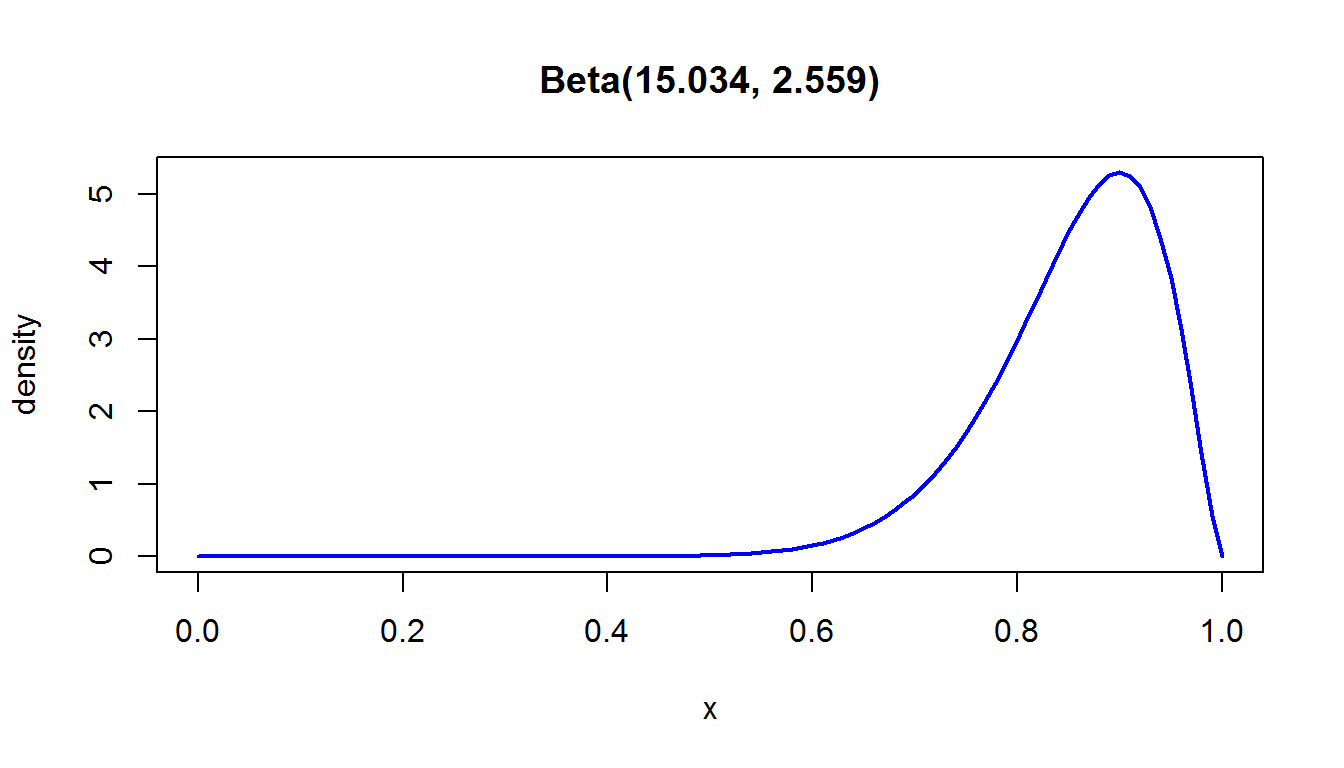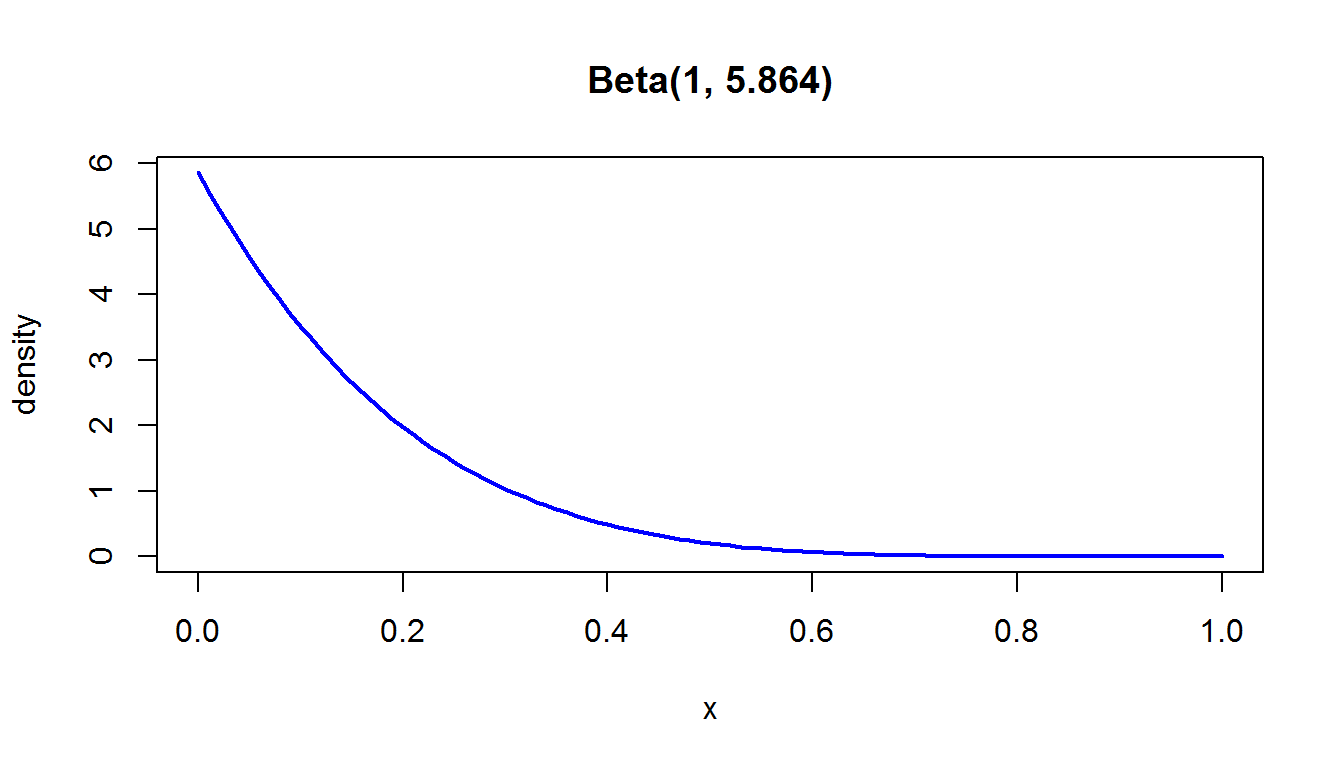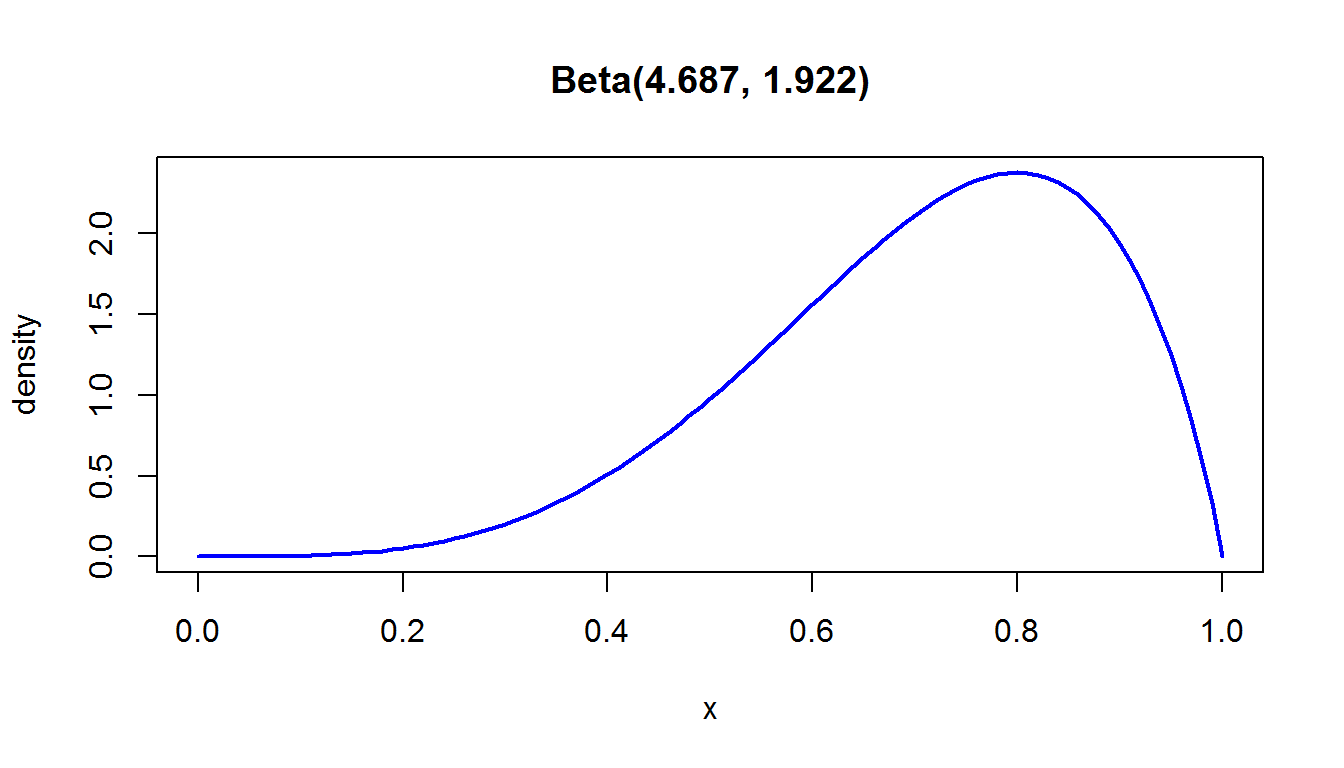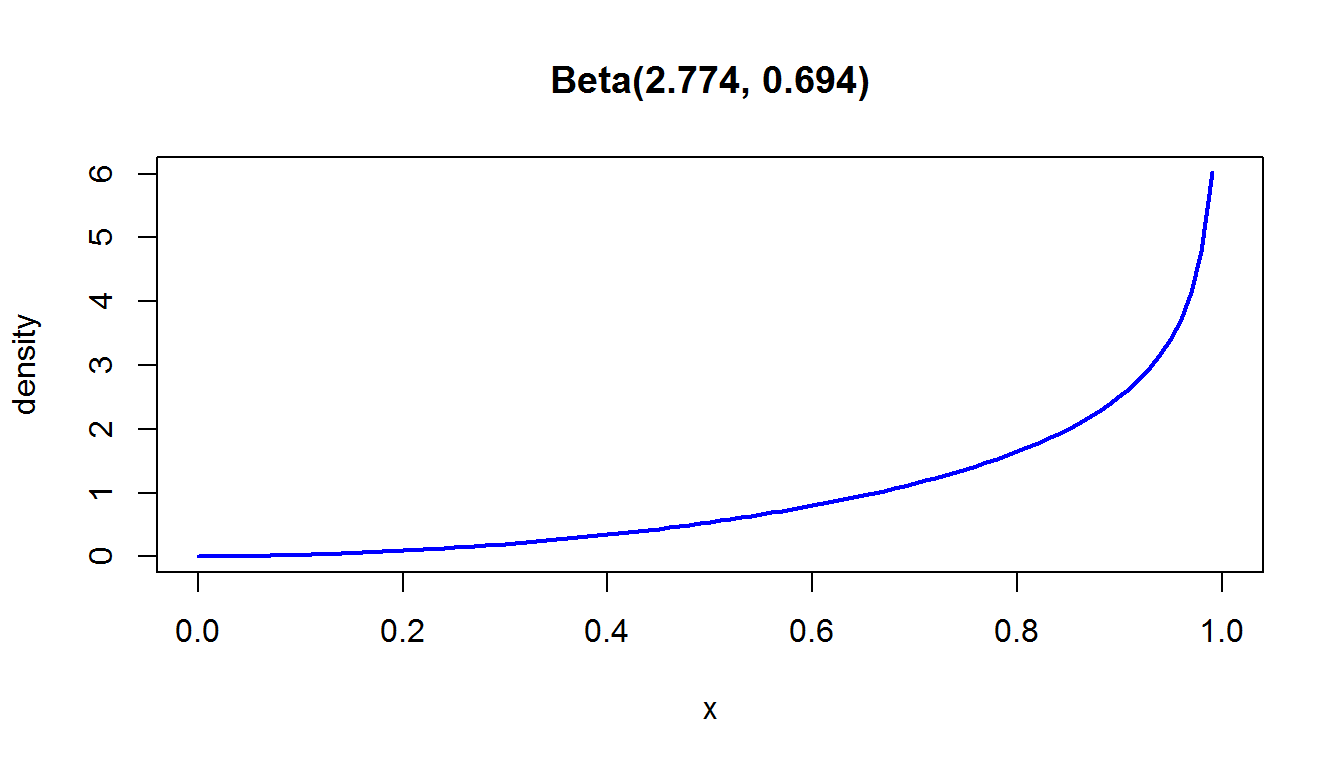the prevalence package
tools for prevalence assessment studies.

## Calculate the parameters of a Beta distribution based on expert information

### Description

The betaExpert function fits a (standard) Beta distribution to expert opinion. The expert provides information on a best-guess estimate (mode or mean), and an uncertainty range:

• The parameter value is with p*100% certainty greater than lower

• The parameter value is with p*100% certainty smaller than upper

• The parameter value lies with p*100% in between lower and upper

### Usage

betaExpert(best, lower, upper, p = 0.95, method = "mode")

## S3 method for class 'betaExpert'
print(x, conf.level = .95, ...)
## S3 method for class 'betaExpert'
plot(x, y, ...)

### Arguments

 best Best-guess estimate; see argument method lower Lower uncertainty limit upper Upper uncertainty limit p Expert’s certainty level method Does best-guess estimate correspond to the mode or to the mean? Defaults to mode x Object of class betaExpert y Currently not implemented conf.level Confidence level used in printing quantiles of resulting Beta distribution ... Other arguments to pass to function print and plot

### Details

The methodology behind the betaExpert function is presented by Branscum et al. (2005) and implemented in the BetaBuster software, written by Chun-Lung Su.

The parameters of a standard Beta distribution are calculated based on a best-guess estimate and a (p)100% uncertainty range, defined by a lower and/or upper limit. The betaExpert function uses minimization (function optimize) to derive $$\alpha$$ and $$\beta$$ from this best guess and lower and/or upper limit. The resulting distribution is a standard 2-parameter Beta distribution: $$Beta(\alpha, \beta)$$.

### Value

A list of class "betaExpert":

 alpha Parameter $$\alpha$$ (shape1) of the Beta distribution beta Parameter $$\beta$$ (shape2) of the Beta distribution

The print method for "betaExpert" additionally calculates the mean, median, mode, variance and range of the corresponding Beta distribution.

Package rriskDistributions, which provides a collection of functions for fitting distributions to given data or by known quantiles.

betaPERT for modelling a generalized Beta distribution based on expert opinion

### Examples

## Most likely value (mode) is 90%
## Expert states with 95% certainty that true value is larger than 70%
betaExpert(best = 0.90, lower = 0.70, p = 0.95)
#>      alpha     beta      mean    median mode         var      2.5%
#> 1 15.03422 2.559357 0.8545289 0.8680246  0.9 0.006685605 0.6616688
#>       97.5%
#> 1 0.9726358

plot(
betaExpert(best = 0.90, lower = 0.70, p = 0.95))## Most likely value (mode) is 0%
## Expert states with 95% certainty that true value is smaller than 40%
plot(
betaExpert(best = 0, upper = 0.40, p = 0.95))## Most likely value (mode) is 80%
## Expert states with 90% certainty that true value lies in between 40% and 90%
plot(
betaExpert(best = 0.80, lower = 0.40, upper = 0.90, p = 0.90))## Mean value is assumed to be 80%
## Expert states with 90% certainty that true value lies in between 40% and 90%
plot(
betaExpert(best = 0.80, lower = 0.40, upper = 0.90, p = 0.90, method = "mean"))